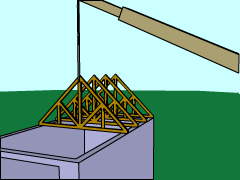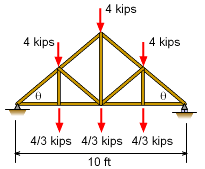Ch 6. Structures Multimedia Engineering Statics 2-D Trusses: Joints 2-D Trusses: Sections 3-D Trusses Frames and Machines
 Chapter 1. Basics 2. Vectors 3. Forces 4. Moments 5. Rigid Bodies 6. Structures 7. Centroids/Inertia 8. Internal Loads 9. Friction 10. Work & Energy Appendix Basic Math Units Sections Search eBooks Dynamics Fluids Math Mechanics Statics Thermodynamics Author(s): Kurt Gramoll ©Kurt GramollSTATICS - CASE STUDY IntroductionProblem Graphic Trusses are commonly used to span distances between two walls. There are many truss designs, but one of the most common a Howe truss due to its simplicity and efficiency. Trusses usually need to carry both roof loads and ceiling loads. Both types of loads are modeled can be modeled as point loads at the truss joints. What is known: The width of the truss is 10 ft. The roof incline angle, θ, is 40°. There are loads at each joint as shown in the diagram at the left Loads are shown in the diagram.Problem Diagram Howe Truss Questions Which member will have the largest tension force and the largest compression force? What is the magnitude of those member forces? Approach Use the method of joints to analyze each pin joint using the basic statics equations, ΣFx = 0 ΣFy = 0 Each joint must be in equilibrium. Only two unknown member forces can be determined per joint. Each member is a two-force member.

Practice Homework and Test problems now available in the 'Eng Statics' mobile app
Includes over 500 problems with complete detailed solutions.
Available now at the Google Play Store and Apple App Store.